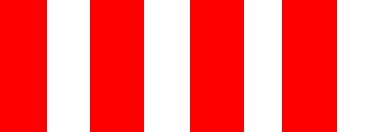#### Howdy, Stranger!

We are about to switch to a new forum software. Until then we have removed the registration on this forum.

# texture sphere : fastest way

edited February 2016

hello all,

all the examples for texture spere are convuleted and old

what is the fastest way for a texture sphere in 3D please?

best, Chrisir ;-)

Tagged:

## Answers

• Fake it. ;)

``````String http = "http://";
PImage img = loadImage(http + "images.clipartpanda.com/sphere-clipart-sphere_Vector_Clipart.png");

void setup(){
size(400,400,P3D);
imageMode(CENTER);
}

void draw(){
background(128);
translate(width/2,height/2,0);
rotateY(map(mouseX,0,width,-PI,PI));
translate(100,0,0);
rotateY(-map(mouseX,0,width,-PI,PI));
image(img,0,0,200,200);
}
``````

:)) :D

• OK, old version - very long

``````/**
* Texture Sphere
* by Gillian Ramsay
*
* Rewritten by Gillian Ramsay to better display the poles.
* Previous version by Mike 'Flux' Chang (and cleaned up by Aaron Koblin).
* Original based on code by Toxi.
*
* A 3D textured sphere with simple rotation control.
*/

int ptsW, ptsH;

PImage img;

int numPointsW;
int numPointsH_2pi;
int numPointsH;

float[] coorX;
float[] coorY;
float[] coorZ;
float[] multXZ;

void setup() {
size(640, 360, P3D);
background(0);
noStroke();
img=loadImage("world32k.jpg");
ptsW=30;
ptsH=30;
// Parameters below are the number of vertices around the width and height
initializeSphere(ptsW, ptsH);
}

// Use arrow keys to change detail settings
void keyPressed() {
if (keyCode == ENTER) saveFrame();
if (keyCode == UP) ptsH++;
if (keyCode == DOWN) ptsH--;
if (keyCode == LEFT) ptsW--;
if (keyCode == RIGHT) ptsW++;
if (ptsW == 0) ptsW = 1;
if (ptsH == 0) ptsH = 2;
// Parameters below are the number of vertices around the width and height
initializeSphere(ptsW, ptsH);
}

void draw() {
background(0);
camera(width/2+map(mouseX, 0, width, -2*width, 2*width),
height/2+map(mouseY, 0, height, -height, height),
height/2/tan(PI*30.0 / 180.0),
width, height/2.0, 0,
0, 1, 0);

pushMatrix();
translate(width/2, height/2, 0);
textureSphere(200, 200, 200, img);
popMatrix();
}

void initializeSphere(int numPtsW, int numPtsH_2pi) {

// The number of points around the width and height
numPointsW=numPtsW+1;
numPointsH_2pi=numPtsH_2pi;  // How many actual pts around the sphere (not just from top to bottom)
numPointsH=ceil((float)numPointsH_2pi/2)+1;  // How many pts from top to bottom (abs(....) b/c of the possibility of an odd numPointsH_2pi)

coorX=new float[numPointsW];   // All the x-coor in a horizontal circle radius 1
coorY=new float[numPointsH];   // All the y-coor in a vertical circle radius 1
coorZ=new float[numPointsW];   // All the z-coor in a horizontal circle radius 1
multXZ=new float[numPointsH];  // The radius of each horizontal circle (that you will multiply with coorX and coorZ)

for (int i=0; i<numPointsW; i++) {  // For all the points around the width
float thetaW=i*2*PI/(numPointsW-1);
coorX[i]=sin(thetaW);
coorZ[i]=cos(thetaW);
}

for (int i=0; i<numPointsH; i++) {  // For all points from top to bottom
if (int(numPointsH_2pi/2) != (float)numPointsH_2pi/2 && i==numPointsH-1) {  // If the numPointsH_2pi is odd and it is at the last pt
float thetaH=(i-1)*2*PI/(numPointsH_2pi);
coorY[i]=cos(PI+thetaH);
multXZ[i]=0;
} else {
//The numPointsH_2pi and 2 below allows there to be a flat bottom if the numPointsH is odd
float thetaH=i*2*PI/(numPointsH_2pi);

//PI+ below makes the top always the point instead of the bottom.
coorY[i]=cos(PI+thetaH);
multXZ[i]=sin(thetaH);
}
}
}

void textureSphere(float rx, float ry, float rz, PImage t) {
// These are so we can map certain parts of the image on to the shape
float changeU=t.width/(float)(numPointsW-1);
float changeV=t.height/(float)(numPointsH-1);
float u=0;  // Width variable for the texture
float v=0;  // Height variable for the texture

beginShape(TRIANGLE_STRIP);
texture(t);
for (int i=0; i<(numPointsH-1); i++) {  // For all the rings but top and bottom
// Goes into the array here instead of loop to save time
float coory=coorY[i];
float cooryPlus=coorY[i+1];

float multxz=multXZ[i];
float multxzPlus=multXZ[i+1];

for (int j=0; j<numPointsW; j++) { // For all the pts in the ring
normal(-coorX[j]*multxz, -coory, -coorZ[j]*multxz);
vertex(coorX[j]*multxz*rx, coory*ry, coorZ[j]*multxz*rz, u, v);
normal(-coorX[j]*multxzPlus, -cooryPlus, -coorZ[j]*multxzPlus);
vertex(coorX[j]*multxzPlus*rx, cooryPlus*ry, coorZ[j]*multxzPlus*rz, u, v+changeV);
u+=changeU;
}
v+=changeV;
u=0;
}
endShape();
}
``````
• new version - nice and lean :

https://www.processing.org/tutorials/pshape/

``````//

PShape globe;

void setup() {
size (1220, 677, OPENGL);
background(0);

PImage img;
img = loadImage("test.jpg");

globe = createShape(SPHERE, 50);
globe.setStroke(false);
globe.setTexture(img);

img=null;
}

void draw() {
//
background(0);
translate(650, 300);

rotateY(map(mouseX, 0, width, 0, TWO_PI));
rotateX(map(mouseY, 0, height, 0, TWO_PI));

shape(globe, 0, 0);
}
//
``````• but thanks, TfGuy44

;-)

• @tfguy44 can you answer so that I can close this ....

it still pops up here....

Thank you

• Huh? What are you asking me to do?

• just what you just did... post here so I can mark your post as answer....

so that the forum stops telling me you have question that now have answers....

thanks, mate!

;-)

Sign In or Register to comment.